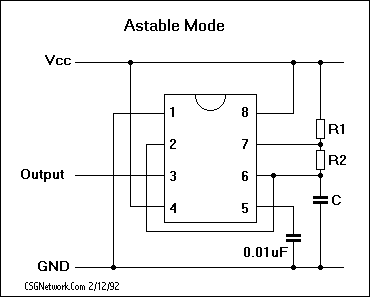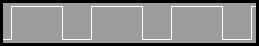# Astable 555 Square Wave Calculator

This calculator requires the use of Javascript enabled and capable browsers. This calculator is designed to give timing values for the 555 timer, based on the control capacitance and resistance. This particular configuration is for an astable square wave calculation. The positive output is high for T(h) seconds based on this formula:
Time High (secs) = 0.693 * (R1 + R2) * C
The negative output is low for T(l) seconds based on this formula:
Time Low (secs) = 0.693 * R2 * C
The frequency is derived by the formula:
Frequency = 1.44 / ((R1 + R2 + R2) * C)
The duty cycle percentage is the relationship of the high time to the overall cycle time and is derived by the formula:
DCP = (T(h) / (T(h) + T(l))) * 100
Where resistance is in ohms and capacitance is in farads. Enter the capacitance in farads (not microfarads) and the resistance in ohms for each resistor. Click on Calculate to return the time high in seconds, the time low in seconds, the duty cycle percentage and the frequency in hertz. You might also wish to see our Monostable 555 Timeout Calculator.555 Calculator Required Data Entry Farads Resistor 1 Resistor 2 Calculated Actual Results Duty Cycle Percentage Frequency In Hertz Seconds Low Seconds High Calculated Rounded Results Duty Cycle Percentage Frequency In Hertz Seconds Low Seconds High
Updated 8.12.11

 Leave us a question or comment on FacebookSearch or Browse Our Site